服装CAD制版系统中曲线绘制自动化方法研究Research on Automatic Method of Curves Drawing in Garment CAD Pattern System

• 全文下载: PDF(1001KB)    PP.9-18   DOI: 10.12677/CSA.2019.91002
• 下载量: 182  浏览量: 242   科研立项经费支持

In the garment CAD pattern system, the garment structure curve was manually drawn by the pat-tern maker and adjusted by his experience and design principles. Each curve took several operations to generate. Bézier curves and NURBS curves based curve drawing methods need to calculate control points. These control points were not on the curve and were computationally complex. In order to make curve drawing not depend on the manual operations of the pattern maker, by studying the drawing process of garment structure curve, an automatic method of curve drawing based on control points of Bézier curves was proposed. The method only relies on several fixed points of the curve and can calculate the control points of the curve automatically; the fixed points are obtained through the design principle of the garment structure curve. Thus, garment structure curve drawing automation is realized; the method can be used for garment CAD pattern system based on parameters design to improve the efficiency of garment patterning.

1. 引言

2. 相关工作

3. 服装结构曲线拟合

3.1. 服装结构曲线特征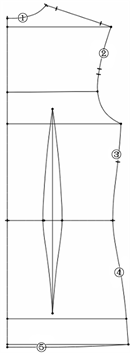Figure 1. Back plate of the shirtFigure 2. Back plate of the shirt layout with pattern process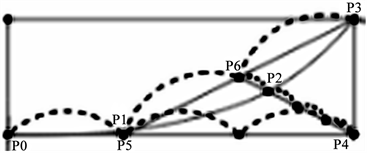Figure 3. Collar arc layout with pattern process

3.2. 服装版型结构常用曲线

3.2.1. 贝塞尔曲线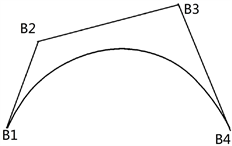(a) C型曲线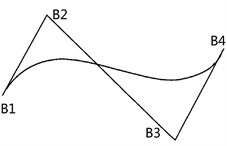(b) S型曲线

Figure 4. Shape of Bézier curve

3.2.2. NURBS曲线 

4. 贝塞尔曲线的控制点计算方法

$B\left(t\right)={\left(1-t\right)}^{3}{B}_{0}+3t{\left(1-t\right)}^{2}{B}_{1}+3{t}^{2}\left(1-t\right){B}_{2}+{t}^{3}{B}_{3},0\le t\le 1$ (1)

4.1. 基于梯度下降法的控制点计算方法

${x}_{0}^{n+1}={x}_{0}^{n}-\alpha \nabla f\left({x}_{0}^{n}\right),n\ge 0$ (2)

${X}_{B}\left(t\right)={\left(1-t\right)}^{3}{x}_{B0}+3t{\left(1-t\right)}^{2}{x}_{B1}+3{t}^{2}\left(1-t\right){x}_{B2}+{t}^{3}{x}_{B3},0\le t\le 1$ (3)

$\nabla {X}_{B}\left({x}_{B1}\right)=\frac{\partial {X}_{B}\left(t\right)}{\partial {x}_{B1}}=\underset{i=0}{\overset{100}{\sum }}\left({x}_{i}-{x}_{bi}\right)3t{\left(1-t\right)}^{2}$ (4)

 (5)

4.2. 基于解方程组法的控制点计算方法

1) 传统方法

$\left\{\begin{array}{l}{Q}_{0}={B}_{0}\\ \cdots \\ {Q}_{i}={C}_{n}^{0}{\left(1-{t}_{i}\right)}^{n}{B}_{0}+{C}_{n}^{1}\left({t}_{i}\right){\left(1-{t}_{i}\right)}^{n-1}{B}_{1}+\cdots +{C}_{n}^{n-1}{\left({t}_{i}\right)}^{n-1}\left(1-{t}_{i}\right){B}_{n-1}+{C}_{n}^{n}{\left({t}_{i}\right)}^{n}{B}_{n}\\ \cdots \\ {Q}_{n}={B}_{n}\end{array}$ (6)

2) 改进的解方程组法

$\left\{\begin{array}{l}{Q}_{0}{}^{\prime }={B}_{0}\\ \cdots \\ {Q}_{i}{}^{\prime }={C}_{n}^{0}{\left(1-\frac{i}{n}\right)}^{n}{B}_{0}+{C}_{n}^{1}\left(\frac{i}{n}\right){\left(1-\frac{i}{n}\right)}^{n-1}{B}_{1}+\cdots +{C}_{n}^{n-1}{\left(\frac{i}{n}\right)}^{n-1}\left(1-\frac{i}{n}\right){B}_{n-1}+{C}_{n}^{n}{\left(\frac{i}{n}\right)}^{n}{B}_{n}\\ \cdots \\ {Q}_{n}{}^{\prime }={B}_{n}\end{array}$ (7)

 (8)

5. 曲线绘制实验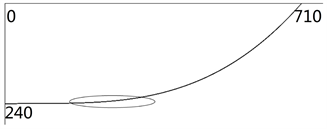Figure 5. Linear fitted curves

5.1. 贝塞尔曲线的控制点计算和绘制Table 1. Calculation results of Bézier curve control point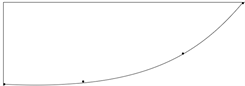(a) 曲线编号1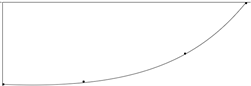(b) 曲线编号2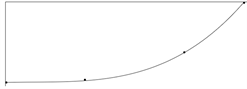(c) 曲线编号3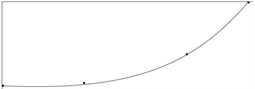(d) 曲线编号4

Figure 6. Linear fitted curvesFigure 7. Linear fitted curves

5.2. 曲线的误差分析

5.3. 曲线绘制自动化的应用Table 3. Parameters of shirt back plate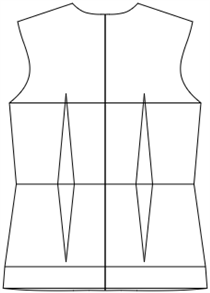Figure 8. Linear fitted curves

6. 结论

  刘筱华, 李尚柏, 黄宁, 等. 曲线算法及其在服装CAD中的应用[J]. 四川联合大学学报(工程科学版), 1997, 1(6): 82-90.  刘卉, 许端清, 陈纯, 等. 服装CAD综述[J]. 计算机辅助设计与图形学学报, 2000, 12(6): 473-479.  滕洪军. 服装CAD样板系统的应用现状及发展[J]. 针织工业, 2011(12): 53-54.  蔡晓媚. 服装CAD制版的智能化探讨[J]. 艺术科技, 2016(11): 93.  施法中. 计算机辅助几何设计与非均匀有理B样条(修订版) [M]. 北京: 高等教育出版社, 2013: 113-135.  张鸿志. 服装CAD中的曲线与曲面及其适用性研究[J]. 浙江纺织服装职业技术学院学报, 2006, 12(4): 29-31.  李重, 马利庄, 黄健. 三次Bezier样条曲线在服装纸样设计中的应用[J]. 纺织学报, 2006, 27(5): 53-55.  王姝. 服装生产优化排料信息系统研究与应用[D]: [硕士学位论文]. 泉州: 华侨大学, 2011: 11-26.  张建桥. 服装CAD曲线表示方法的研究[J]. 纺织高校基础科学学报, 2010, 23(3): 382-385.  宋琨, 张渭源. 用贝塞尔曲线拟合服装结构曲线的处理方法[J]. 国际纺织导报, 2001(4): 77-79.  马华, 刘峰, 任春丽. Bezier曲线的计算机实现[J]. 西安电子科技大学学报(自然科学版), 2002, 29(4): 565-568.  刘勇奎. Bezier曲线的快速绘制方法[J]. 微计算机应用, 1994, 3(5): 34-35.  邓达. 三次Bezier曲线的圆弧样条逼近[J]. 重庆工学院学报(自然科学版), 2007, 21(7): 144-146.  刘庚鑫. NURBS曲线在服装原型中的应用[J]. 广西纺织科技, 2007, 36(2): 34-37.  (美)John H. Mathews. 数值方法(MATLAB版) [M]. 第4版. 北京: 电子工业出版社, 2005: 196-207.